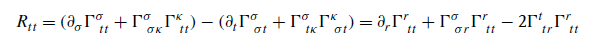# Ricci Tensor Equation in Zee's "Einstein's Gravity in a Nutshell" Explained

• Lapidus

#### Lapidus

In Zee's "Einstein's Gravity in a Nutshell" on page 363, while deriving the Schwarzschild solution, we haveHow does it work? How are the rhs and lhs equal? Where does the factor 2 come from, why just one derivative left?

thanks for any replies!

The solution is assumed independent of the t-coordinate so derivatives wrt that coordinate vanish. The 2 appears because you have the possibilities if sigma and kappa taking values r and t in tha term. This can be done in two ways.

•Lapidus